# x86/x64 AESNI Instructions

As for AES cipher, FIPS 197 issued by the government agency of the United States, is the original specification.

## Key length, Block Length, Number of Rounds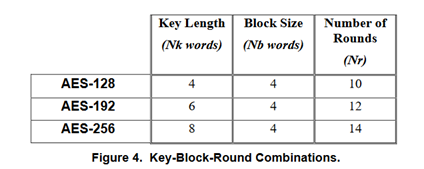(from FIPS 197)

## state array

The state array is a 2-dimensional array of 4 * 4 bytes. The 16-byte plaintext is put here, and processed by repeating the procedure called "round", to generate the ciphertext. Rounds are repeated for Nr times.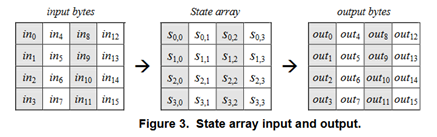(from FIPS 197)

AESNI instructions handle the whole state array in one XMMWORD.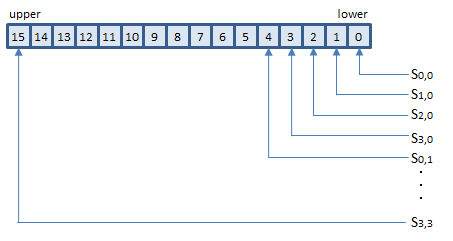Note that no byte-order swapping (endian conversion) required for using AESNI instructions.

## w array

The w array is a one-dimensional array of 4 * (Nr + 1) words. (An AES "word" is 32-bit). Before encryption, the Nk-word cipher key must be expanded to the w array by the procedure called KeyExpansion. In each round, 4 words of the w array are consumed. Before the rounds, 4 words are consumed in pre-processing, so 4 * (Nr + 1) words are required.

AESNI instructions handle 4 words of the w array (for one round) in one XMMWORD.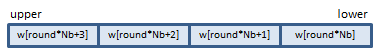# Encryption

The following is the AES encryption algorithm defined in FIPS 197. AESNI instructions do A and B. C is just an XOR operation, so PXOR can do it.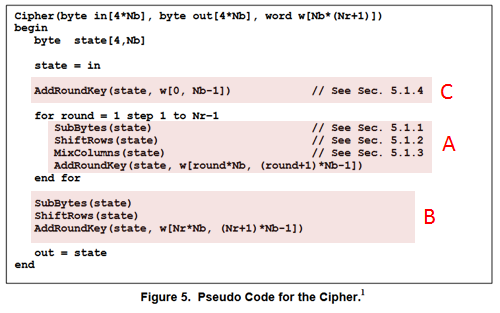## AESENC - AES ENCryptAESENCLAST - AES ENCrypt LAST

AESENC xmm1, xmm2/m128
__m128i  _mm_aesenc_si128(__m128i state, __m128i w);
AESENCLAST xmm1, xmm2/m128
__m128i  _mm_aesenclast_si128(__m128i state, __m128i w);VAESENC xmm1, xmm2, xmm3/m128
VAESENCLAST xmm1, xmm2, xmm3/m128AESENC instruction processes A above (one round).

B is the last round. AESENCLAST instruction processes this round.

Inputs: (1) = the state array before the round, (2) = the w array elements for the round.

Output: (3) = the state array after the round

# Generating the w array

Before the encryption, the w array must be generated by the KeyExpansion procedure defined in FIPS 197 as following.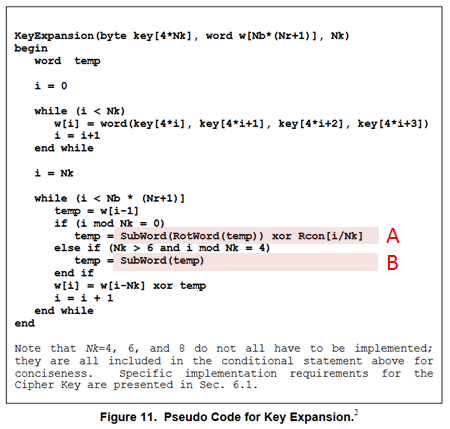Rcon is an array of constants calculated by the method defined in FIPS 197. The values are as following.

static const BYTE Rcon[] = {
0x8d, 0x01, 0x02, 0x04, 0x08, 0x10, 0x20, 0x40, 0x80, 0x1b, 0x36, 0x6c, 0xd8, 0xab, 0x4d, 0x9a,
};

Only elements  to  are actually used in the AES cipher.

Note that no byte-order swapping required when copying the key to the w array.

## AESKEYGENASSIST - AES KEY GENeration ASSIST

AESKEYGENASSIST xmm1, xmm2/m128, imm8
VAESKEYGENASSIST xmm1, xmm2/m128, imm8
__m128i  _mm_aeskeygenassist_si128(__m128i temp, const int Rcon);AESKEYGENASSIST instruction calculates the values of A and B expressions.

Inputs: (1) = value of temp, (2) = Rcon[i / Nk]

Output: (3) = the values calculated

# Decryption

FIPS 197 defines 2 algorithms of the AES decryption: InvCipher and EqInvCipher. Both algorithms produce the same result, but only the latter is supported by AESNI instructions.

EqInvCipher uses the dw array, which is generated from the w array with some additional procedure. How to generate the dw array is described later.## AESDEC - AES DECryptAESDECLAST - AES DECrypt LAST

AESDEC xmm1, xmm2/m128
__m128i  _mm_aesdec_si128(__m128i state, __m128i dw);
AESDECLAST xmm1, xmm2/m128
__m128i  _mm_aesdeclast_si128(__m128i state, __m128i dw);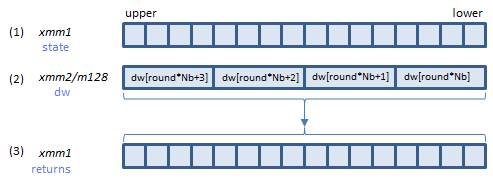VAESDEC xmm1, xmm2, xmm3/m128
VAESDECLAST xmm1, xmm2, xmm3/m128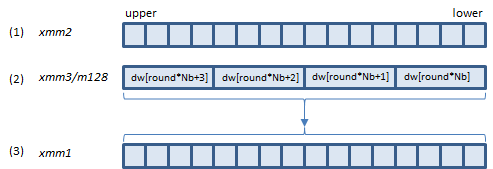AESDEC instruction processes A above (one round).

B is the last round. AESDECLAST instruction processes this round.

Inputs: (1) = the state array before the round, (2) = the dw array elements for the round.

Output: (3) = the state array after the round

# Generating the dw array

The dw array for EqInvCipher is generated from the w array with the following additional process.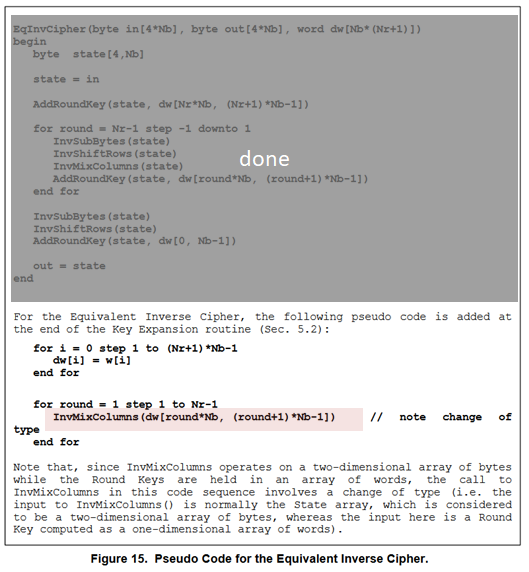## AESIMC - AES InvMixColumns

AESIMC xmm1, xmm2/m128
VAESIMC xmm1, xmm2/m128
__m128i  _mm_aesimc_si128(__m128i w);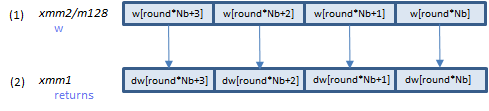AESIMC instruction processes the InvMixColumns() function above.

Input: (1) = the w array elements.

Output: (2) = the dw array elements.

# Samples

AES-128   AES-192   AES-256

```#pragma once

#include <intrin.h>

class AES128_NI
{
public:
// AES128
static const int Nk = 4;
static const int Nb = 4;
static const int Nr = 10;

protected:
__m128i w128[Nr + 1];
__m128i dw128[Nr + 1];
bool decrypting;

public:
AES128_NI(const unsigned char key[4 * Nk], bool decrypting)
: decrypting(decrypting)
{
KeyExpansion(key);
}

void Cipher(const unsigned char in[4 * Nb], unsigned char out[4 * Nb]);
void EqInvCipher(const unsigned char in[4 * Nb], unsigned char out[4 * Nb]);

protected:
void KeyExpansion(const unsigned char key[4 * Nk]);
};
```

```#include "AES128_NI.h"

//Cipher(byte in[4*Nb], byte out[4*Nb], word w[Nb*(Nr+1)])
//begin
void AES128_NI::Cipher(const unsigned char in[4 * Nb], unsigned char out[4 * Nb])
{
//  ASSERT(!decrypting);

//state = in
__m128i state = _mm_loadu_si128(reinterpret_cast<const __m128i *>(in));

// just XOR
state = _mm_xor_si128(state, w128);

//for round = 1 step 1 to Nr-1
//SubBytes(state)
//ShiftRows(state)
//MixColumns(state)
//end for
state = _mm_aesenc_si128(state, w128);
state = _mm_aesenc_si128(state, w128);
state = _mm_aesenc_si128(state, w128);
state = _mm_aesenc_si128(state, w128);
state = _mm_aesenc_si128(state, w128);
state = _mm_aesenc_si128(state, w128);
state = _mm_aesenc_si128(state, w128);
state = _mm_aesenc_si128(state, w128);
state = _mm_aesenc_si128(state, w128);

// The last round
//SubBytes(state)
//ShiftRows(state)
state = _mm_aesenclast_si128(state, w128[Nr]);

//out = state
_mm_storeu_si128(reinterpret_cast<__m128i *>(out), state);

//end
}

//EqInvCipher(byte in[4*Nb], byte out[4*Nb], word dw[Nb*(Nr+1)])
//begin
void AES128_NI::EqInvCipher(const unsigned char in[4 * Nb], unsigned char out[4 * Nb])
{
//  ASSERT(decrypting);

//byte  state[4,Nb]
//state = in
__m128i state = _mm_loadu_si128(reinterpret_cast<const __m128i *>(in));

state = _mm_xor_si128(state, w128[Nr]);

//for round = Nr-1 step -1 downto 1
//InvSubBytes(state)
//InvShiftRows(state)
//InvMixColumns(state)
//end for
state = _mm_aesdec_si128(state, dw128);
state = _mm_aesdec_si128(state, dw128);
state = _mm_aesdec_si128(state, dw128);
state = _mm_aesdec_si128(state, dw128);
state = _mm_aesdec_si128(state, dw128);
state = _mm_aesdec_si128(state, dw128);
state = _mm_aesdec_si128(state, dw128);
state = _mm_aesdec_si128(state, dw128);
state = _mm_aesdec_si128(state, dw128);

//InvSubBytes(state)
//InvShiftRows(state)
state = _mm_aesdeclast_si128(state, dw128);

//out = state
_mm_storeu_si128(reinterpret_cast<__m128i *>(out), state);
//end
}

//static const BYTE Rcon[] = {
//  0x8d, 0x01, 0x02, 0x04, 0x08, 0x10, 0x20, 0x40, 0x80, 0x1b, 0x36, 0x6c, 0xd8, 0xab, 0x4d, 0x9a,
//};

//KeyExpansion(byte key[4*Nk], word w[Nb*(Nr+1)], Nk)
//begin
void AES128_NI::KeyExpansion(const unsigned char key[4 * Nk])
{
//word  temp
//i = 0

//while (i < Nk)
//w[i] = word(key[4*i], key[4*i+1], key[4*i+2], key[4*i+3])
//i = i+1
//end while
__m128i work = _mm_loadu_si128(reinterpret_cast<const __m128i*>(key));  // work = w3 : w2 : w1 : w0
w128 = work;

//i = Nk
//while (i < Nb * (Nr+1)]
//temp = w[i-1]
//if (i mod Nk = 0)
//temp = SubWord(RotWord(temp)) xor Rcon[i/Nk]
//else if (Nk > 6 and i mod Nk = 4)
//temp = SubWord(temp)
//end if
//w[i] = w[i-Nk] xor temp
//i = i + 1
//end while

__m128i t, t2;

// f(w) = SubWord(RotWord(w)) xor Rcon

// work = w3 : w2 : w1 : w0
// w4 = w0^f(w3)
// w5 = w1^w4 = w1^w0^f(w3)
// w6 = w2^w5 = w2^w1^w0^f(w3)
// w7 = w3^w6 = w3^w2^w1^w0^f(w3)
#define EXPAND(n, RCON) \
t  = _mm_slli_si128(work, 4);       /* t    = w2                : w1             : w0          : 0        */\
t  = _mm_xor_si128(work, t);        /* t    = w3^w2             : w2^w1          : w1^w0       : w0       */\
t2 = _mm_slli_si128(t, 8);          /* t2   = w1^w0             : w0             : 0           : 0        */\
t  = _mm_xor_si128(t, t2);          /* t    = w3^w2^w1^w0       : w2^w1^w0       : w1^w0       : w0       */\
work = _mm_aeskeygenassist_si128(work, RCON);           /* work = f(w3) : - : - : - */                      \
work = _mm_shuffle_epi32(work, 0xFF);/*work = f(w3)             : f(w3)          : f(w3)       ; f(w3)    */\
work = _mm_xor_si128(t, work);      /* work = w3^w2^w1^w0^f(w3) : w2^w1^w0^f(w3) : w1^w0^f(w3) : w0^f(w3) */\
w128[n] = work;                     /* work = w7 : w6 : w5 : w4 */

EXPAND(1, 0x01);

// Go on...
EXPAND(2, 0x02);
EXPAND(3, 0x04);
EXPAND(4, 0x08);
EXPAND(5, 0x10);
EXPAND(6, 0x20);
EXPAND(7, 0x40);
EXPAND(8, 0x80);
EXPAND(9, 0x1b);
EXPAND(10, 0x36);

if (decrypting) {
//for i = 0 step 1 to (Nr+1)*Nb-1
//dw[i] = w[i]
//end for
dw128 = w128;

//for round = 1 step 1 to Nr-1
//InvMixColumns(dw[round*Nb, (round+1)*Nb-1])
//end for
dw128 = _mm_aesimc_si128(w128);
dw128 = _mm_aesimc_si128(w128);
dw128 = _mm_aesimc_si128(w128);
dw128 = _mm_aesimc_si128(w128);
dw128 = _mm_aesimc_si128(w128);
dw128 = _mm_aesimc_si128(w128);
dw128 = _mm_aesimc_si128(w128);
dw128 = _mm_aesimc_si128(w128);
dw128 = _mm_aesimc_si128(w128);

dw128[Nr] = w128[Nr];
}
//end
}
```
```#pragma once

#include <intrin.h>

class AES192_NI
{
public:
// AES192
static const int Nk = 6;
static const int Nb = 4;
static const int Nr = 12;

protected:
__m128i w128[Nr + 1];
__m128i dw128[Nr + 1];
bool decrypting;

public:
AES192_NI(const unsigned char key[4 * Nk], bool decrypting)
: decrypting(decrypting)
{
KeyExpansion(key);
}

void Cipher(const unsigned char in[4 * Nb], unsigned char out[4 * Nb]);
void EqInvCipher(const unsigned char in[4 * Nb], unsigned char out[4 * Nb]);

protected:
void KeyExpansion(const unsigned char key[4 * Nk]);
};
```

```#include "AES192_NI.h"

//Cipher(byte in[4*Nb], byte out[4*Nb], word w[Nb*(Nr+1)])
//begin
void AES192_NI::Cipher(const unsigned char in[4 * Nb], unsigned char out[4 * Nb])
{
//  ASSERT(!decrypting);

//state = in

// just XOR
state = _mm_xor_si128(state, w128);

//for round = 1 step 1 to Nr-1
//SubBytes(state)
//ShiftRows(state)
//MixColumns(state)
//end for
state = _mm_aesenc_si128(state, w128);
state = _mm_aesenc_si128(state, w128);
state = _mm_aesenc_si128(state, w128);
state = _mm_aesenc_si128(state, w128);
state = _mm_aesenc_si128(state, w128);
state = _mm_aesenc_si128(state, w128);
state = _mm_aesenc_si128(state, w128);
state = _mm_aesenc_si128(state, w128);
state = _mm_aesenc_si128(state, w128);
state = _mm_aesenc_si128(state, w128);
state = _mm_aesenc_si128(state, w128);

// The last round
//SubBytes(state)
//ShiftRows(state)
state = _mm_aesenclast_si128(state, w128[Nr]);

//out = state
_mm_storeu_si128(reinterpret_cast<__m128i*>(out), state);

//end
}

//EqInvCipher(byte in[4*Nb], byte out[4*Nb], word dw[Nb*(Nr+1)])
//begin
void AES192_NI::EqInvCipher(const unsigned char in[4 * Nb], unsigned char out[4 * Nb])
{
//  ASSERT(decrypting);

//byte  state[4,Nb]
//state = in

state = _mm_xor_si128(state, dw128[Nr]);

//for round = Nr-1 step -1 downto 1
//InvSubBytes(state)
//InvShiftRows(state)
//InvMixColumns(state)
//end for
state = _mm_aesdec_si128(state, dw128);
state = _mm_aesdec_si128(state, dw128);
state = _mm_aesdec_si128(state, dw128);
state = _mm_aesdec_si128(state, dw128);
state = _mm_aesdec_si128(state, dw128);
state = _mm_aesdec_si128(state, dw128);
state = _mm_aesdec_si128(state, dw128);
state = _mm_aesdec_si128(state, dw128);
state = _mm_aesdec_si128(state, dw128);
state = _mm_aesdec_si128(state, dw128);
state = _mm_aesdec_si128(state, dw128);

//InvSubBytes(state)
//InvShiftRows(state)
state = _mm_aesdeclast_si128(state, dw128);

//out = state
_mm_storeu_si128(reinterpret_cast<__m128i*>(out), state);
//end
}

//static const BYTE Rcon[] = {
//  0x8d, 0x01, 0x02, 0x04, 0x08, 0x10, 0x20, 0x40, 0x80, 0x1b, 0x36, 0x6c, 0xd8, 0xab, 0x4d, 0x9a,
//};

//KeyExpansion(byte key[4*Nk], word w[Nb*(Nr+1)], Nk)
//begin
void AES192_NI::KeyExpansion(const unsigned char key[4 * Nk])
{
//word  temp
//i = 0

//while (i < Nk)
//w[i] = word(key[4*i], key[4*i+1], key[4*i+2], key[4*i+3])
//i = i+1
//end while
__m128i work1 = _mm_loadu_si128(reinterpret_cast<const __m128i*>(key));         // w3 : w2 : w1 : w0
__m128i work2 = _mm_loadl_epi64(reinterpret_cast<const __m128i*>(key + 16));    // - : - : w5 : w4

w128 = work1;

//i = Nk
//while (i < Nb * (Nr+1)]
//temp = w[i-1]
//if (i mod Nk = 0)
//temp = SubWord(RotWord(temp)) xor Rcon[i/Nk]
//else if (Nk > 6 and i mod Nk = 4)
//temp = SubWord(temp)
//end if
//w[i] = w[i-Nk] xor temp
//i = i + 1
//end while

__m128i t, t2;
__m128i work3;

// f(w) = SubWord(RotWord(w)) xor Rcon

// work1 = w3 : w2 : w1 : w0
// work2 = - : - : w5 : w4
// w6 = w0^f(w5)
// w7 = w1^w6 = w1^w0^f(w5)
t = _mm_aeskeygenassist_si128(work2, 0x01); /* t = - : - : f(w5) : -     */
t = _mm_shuffle_epi32(t, 0x55);     /* t     = f(w5) : f(w5) : f(w5) : f(w5) */
t2 = _mm_slli_si128(work1, 4);      /* t2    = -     : -     : w0    : 0     */
t2 = _mm_xor_si128(work1, t2);      /* t2    = -     : -     : w1^w0 : w0    */
t = _mm_xor_si128(t2, t);           /* t     = -     : -     : w7    : w6    */
work2 = _mm_unpacklo_epi64(work2, t);   /* work2 = w7    : w6    : w5    : w4    */
w128 = work2;

// work1 = w3 : w2 : w1 : w0
// work2 = w7 : w6 : w5 : w4
// w8 = w2^w7
// w9 = w3^w8 = w3^w2^w7
// w10 = w4^w9 = w4^w3^w2^w7
// w11 = w5^w10 = w5^w4^w3^w2^w7
#define EXPAND1(n)                                                                           \
t     = _mm_alignr_epi8(work2, work1, 8);/* t    = w5          : w4       : w3    : w2 */\
work3 = _mm_shuffle_epi32(work2, 0xFF); /* work3 = w7          : w7       : w7    : w7 */\
t2    = _mm_slli_si128(t, 4);           /* t2    = w4          : w3       : w2    : 0  */\
t     = _mm_xor_si128(t, t2);           /* t     = w5^w4       : w4^w3    : w3^w2 : w2 */\
t2    = _mm_slli_si128(t, 8);           /* t2    = w3^w2       : w2       : 0     : 0  */\
t2    = _mm_xor_si128(t, t2);           /* t2    = w5^w4^w3^w2 : w4^w3^w2 : w3^w2 : w2 */\
work3 = _mm_xor_si128(t2, work3);       /* work3 = w11         : w10      : w9    : w8 */\
w128[n] = work3;

EXPAND1(2);

// work2 = w7 : w6 : w5 : w4
// work3 = w11: w10 : w9 : w8
// w12 = w6^f(w11)
// w13 = w7^w12 = w7^w6^f(w11)
// w14 = w8^w13 = w8^w7^w6^f(w11)
// w15 = w9^w14 = w9^w8^w7^w6^f(w11)
#define EXPAND2(n, RCON)                                                                          \
t     = _mm_alignr_epi8(work3, work2, 8);/*t     = w9          : w8       : w7     : w6     */\
work1 = _mm_aeskeygenassist_si128(work3, RCON);/* work1 = f(w11) : - : - : -                */\
work1 = _mm_shuffle_epi32(work1, 0xFF); /* work1 = f(w11)      : f(w11)   : f(w11) : f(w11) */\
t2    = _mm_slli_si128(t, 4);           /* t2    = w8          : w7       : w6     : 0      */\
t     = _mm_xor_si128(t, t2);           /* t     = w9^w8       : w8^w7    : w7^w6  : w6     */\
t2    = _mm_slli_si128(t, 8);           /* t2    = w7^w6       : w6       : 0      : 0      */\
t     = _mm_xor_si128(t, t2);           /* t     = w9^w8^w7^w6 : w8^w7^w6 : w7^w6  : w6     */\
work1 = _mm_xor_si128(t, work1);        /* work1 = w15         : w14      : w13    : w12    */\
w128[n] = work1;

EXPAND2(3, 0x02);

const __m128i idx1 = _mm_set_epi8(-1, -1, -1, -1, -1, -1, -1, -1, 15, 14, 13, 12, 15, 14, 13, 12);
const __m128i idx2 = _mm_set_epi8(7, 6, 5, 4, 7, 6, 5, 4, -1, -1, -1, -1, -1, -1, -1, -1);

// work3 = w11 : w10 : w9 : w8
// work1 = w15 : w14 : w13 : w12
// w16 = w10^w15
// w17 = w11^w16 = w11^w10^w15
// w18 = w12^f(w17)
// w19 = w13^w18 = w13^w12^f(w17)
#define EXPAND3(n, RCON)                                                                      \
work2 = _mm_alignr_epi8(work1, work3, 8);/*work2 = w13     : w12    : w11     : w10     */\
t     = _mm_slli_epi64(work2, 32);      /* t     = w12     : 0      : w10     : 0       */\
work2 = _mm_xor_si128(work2, t);        /* work2 = w13^w12 : w12    : w11^w10 : w10     */\
t     = _mm_shuffle_epi8(work1, idx1);  /* t     = 0       : 0      : w15     : w15     */\
work2 = _mm_xor_si128(work2, t);        /* work2 = w13^w12 : w12    : w17     : w16     */\
t     = _mm_aeskeygenassist_si128(work2, RCON);/*t=-       : -      : f(w17)  : -       */\
t     = _mm_shuffle_epi8(t, idx2);      /* t     = f(w17)  : f(w17) : 0       : 0       */\
work2 = _mm_xor_si128(work2, t);        /* work2 = w19     : w18    : w17     : w16     */\
w128[n] = work2;

EXPAND3(4, 0x04);

// Go on...
EXPAND1(5);         // w20-w23
EXPAND2(6, 0x08);   // w24-w27
EXPAND3(7, 0x10);   // w28-w31

EXPAND1(8);         // w32-w35
EXPAND2(9, 0x20);   // w36-w39
EXPAND3(10, 0x40);  // w40-w43

EXPAND1(11);        // w44-w47
EXPAND2(12, 0x80);  // w48-w51

if (decrypting) {
//for i = 0 step 1 to (Nr+1)*Nb-1
//dw[i] = w[i]
//end for
dw128 = w128;

//for round = 1 step 1 to Nr-1
//InvMixColumns(dw[round*Nb, (round+1)*Nb-1])
//end for
dw128 = _mm_aesimc_si128(w128);
dw128 = _mm_aesimc_si128(w128);
dw128 = _mm_aesimc_si128(w128);
dw128 = _mm_aesimc_si128(w128);
dw128 = _mm_aesimc_si128(w128);
dw128 = _mm_aesimc_si128(w128);
dw128 = _mm_aesimc_si128(w128);
dw128 = _mm_aesimc_si128(w128);
dw128 = _mm_aesimc_si128(w128);
dw128 = _mm_aesimc_si128(w128);
dw128 = _mm_aesimc_si128(w128);

dw128[Nr] = w128[Nr];
}
//end
}
```
```#pragma once

#include <intrin.h>

class AES256_NI
{
public:
// AES256
static const int Nk = 8;
static const int Nb = 4;
static const int Nr = 14;

protected:
__m128i w128[Nr + 1];
__m128i dw128[Nr + 1];
bool decrypting;

public:
AES256_NI(const unsigned char key[4 * Nk], bool decrypting)
: decrypting(decrypting)
{
KeyExpansion(key);
}

void Cipher(const unsigned char in[4 * Nb], unsigned char out[4 * Nb]);
void EqInvCipher(const unsigned char in[4 * Nb], unsigned char out[4 * Nb]);

protected:
void KeyExpansion(const unsigned char key[4 * Nk]);
};
```

```#include "AES256_NI.h"

//Cipher(byte in[4*Nb], byte out[4*Nb], word w[Nb*(Nr+1)])
//begin
void AES256_NI::Cipher(const unsigned char in[4 * Nb], unsigned char out[4 * Nb])
{
//  ASSERT(!decrypting);

//state = in
__m128i state = _mm_loadu_si128(reinterpret_cast<const __m128i *>(in));

// Just XOR
state = _mm_xor_si128(state, w128);

//for round = 1 step 1 to Nr-1
//SubBytes(state)
//ShiftRows(state)
//MixColumns(state)
//end for
state = _mm_aesenc_si128(state, w128);
state = _mm_aesenc_si128(state, w128);
state = _mm_aesenc_si128(state, w128);
state = _mm_aesenc_si128(state, w128);
state = _mm_aesenc_si128(state, w128);
state = _mm_aesenc_si128(state, w128);
state = _mm_aesenc_si128(state, w128);
state = _mm_aesenc_si128(state, w128);
state = _mm_aesenc_si128(state, w128);
state = _mm_aesenc_si128(state, w128);
state = _mm_aesenc_si128(state, w128);
state = _mm_aesenc_si128(state, w128);
state = _mm_aesenc_si128(state, w128);

// The last round
//SubBytes(state)
//ShiftRows(state)
state = _mm_aesenclast_si128(state, w128[Nr]);

//out = state
_mm_storeu_si128(reinterpret_cast<__m128i *>(out), state);

//end
}

//EqInvCipher(byte in[4*Nb], byte out[4*Nb], word dw[Nb*(Nr+1)])
//begin
void AES256_NI::EqInvCipher(const unsigned char in[4 * Nb], unsigned char out[4 * Nb])
{
//  ASSERT(decrypting);

//byte  state[4,Nb]
//state = in
__m128i state = _mm_loadu_si128(reinterpret_cast<const __m128i *>(in));

state = _mm_xor_si128(state, dw128[Nr]);

//for round = Nr-1 step -1 downto 1
//InvSubBytes(state)
//InvShiftRows(state)
//InvMixColumns(state)
//end for
state = _mm_aesdec_si128(state, dw128);
state = _mm_aesdec_si128(state, dw128);
state = _mm_aesdec_si128(state, dw128);
state = _mm_aesdec_si128(state, dw128);
state = _mm_aesdec_si128(state, dw128);
state = _mm_aesdec_si128(state, dw128);
state = _mm_aesdec_si128(state, dw128);
state = _mm_aesdec_si128(state, dw128);
state = _mm_aesdec_si128(state, dw128);
state = _mm_aesdec_si128(state, dw128);
state = _mm_aesdec_si128(state, dw128);
state = _mm_aesdec_si128(state, dw128);
state = _mm_aesdec_si128(state, dw128);

//InvSubBytes(state)
//InvShiftRows(state)
state = _mm_aesdeclast_si128(state, dw128);

//out = state
_mm_storeu_si128(reinterpret_cast<__m128i *>(out), state);
//end
}

//static const BYTE Rcon[] = {
//  0x8d, 0x01, 0x02, 0x04, 0x08, 0x10, 0x20, 0x40, 0x80, 0x1b, 0x36, 0x6c, 0xd8, 0xab, 0x4d, 0x9a,
//};

//KeyExpansion(byte key[4*Nk], word w[Nb*(Nr+1)], Nk)
//begin
void AES256_NI::KeyExpansion(const unsigned char key[4 * Nk])
{
//word  temp
//i = 0

//while (i < Nk)
//w[i] = word(key[4*i], key[4*i+1], key[4*i+2], key[4*i+3])
//i = i+1
//end while
__m128i work2 = _mm_loadu_si128(reinterpret_cast<const __m128i*>(key + 16));
w128 = work1;    // work1 = w3 : w2 : w1 : w0
w128 = work2;    // work2 = w7 : w6 : w5 : w4

//i = Nk
//while (i < Nb * (Nr+1)]
//temp = w[i-1]
//if (i mod Nk = 0)
//temp = SubWord(RotWord(temp)) xor Rcon[i/Nk]
//else if (Nk > 6 and i mod Nk = 4)
//temp = SubWord(temp)
//end if
//w[i] = w[i-Nk] xor temp
//i = i + 1
//end while

__m128i t;

// f(w) = SubWord(RotWord(w)) xor Rcon
// g(w) = SubWord(w)

// work1 = w3 : w2 : w1 : w0
// work2 = w7 : w6 : w5 : w4
// w8 = w0^f(w7)
// w9 = w1^w8 = w1^w0^f(w7)
// w10 = w2^w9 = w2^w1^w0^f(w7)
// w11 = w3^w11 = w3^w2^w1^w0^f(w7)
#define EXPAND1(n, RCON)                                                                                        \
t = _mm_slli_si128(work1, 4);   /* t     = w2                : w1             : w0          : 0        */   \
work1 = _mm_xor_si128(work1, t);/* work1 = w3^w2             : w2^w1          : w1^w0       : w0       */   \
t = _mm_slli_si128(work1, 8);   /* t     = w1^w0             : w0             : 0           : 0        */   \
work1 = _mm_xor_si128(work1, t);/* work1 = w3^w2^w1^w0       : w2^w1^w0       : w1^w0       : w0       */   \
t = _mm_aeskeygenassist_si128(work2, RCON); /* t = f(w7) : - : - : - */                                     \
t = _mm_shuffle_epi32(t, 0xFF); /* t     = f(w7)             : f(w7)          : f(w7)       : f(w7)    */   \
work1 = _mm_xor_si128(work1, t);/* work1 = w3^w2^w1^w0^f(w7) : w2^w1^w0^f(w7) : w1^w0^f(w7) : w0^f(w7) */   \
w128[n] = work1;                /* work1 = w11 : w10 : w9 : w8 */

EXPAND1(2, 0x01);

// work2 = w7 : w6 : w5 : w4
// work1 = w11 : w10 : w9 : w8
// w12 = w4^g(w11)
// w13 = w5^w12 = w5^w4^g(w11)
// w14 = w6^w13 = w6^w5^w4^g(w11)
// w15 = w7^w14 = w7^w6^w5^w4^g(w11)
#define EXPAND2(n)                                                                                              \
t = _mm_slli_si128(work2, 4);   /* t     = w6                : w5             : w4          : 0        */   \
work2 = _mm_xor_si128(work2, t);/* work2 = w7^w6             : w6^w5          : w5^w4       : w4       */   \
t = _mm_slli_si128(work2, 8);   /* t     = w5^w4             : w4             : 0           : 0        */   \
work2 = _mm_xor_si128(work2, t);/* work2 = w7^w6^w5^w4       : w6^w5^w4       : w5^w4       : w4       */   \
t = _mm_aeskeygenassist_si128(work1, 0);    /* t = - : g(w11) : - : - */                                    \
t = _mm_shuffle_epi32(t, 0xAA); /* t     = g(w11)            : g(w11)         : g(w11)      : g(w11)   */   \
work2 = _mm_xor_si128(work2, t);/* work2 = w7^w6^w5^w4^g(w11): w6^w5^w4^g(w11): w5^w4^g(w11): w4^g(w11)*/   \
w128[n] = work2;                /* work2 = w15 : w14 : w13 : w12 */

EXPAND2(3);

// Go on...
EXPAND1(4, 0x02);
EXPAND2(5);
EXPAND1(6, 0x04);
EXPAND2(7);
EXPAND1(8, 0x08);
EXPAND2(9);
EXPAND1(10, 0x10);
EXPAND2(11);
EXPAND1(12, 0x20);
EXPAND2(13);
EXPAND1(14, 0x40);

if (decrypting) {
//for i = 0 step 1 to (Nr+1)*Nb-1
//dw[i] = w[i]
//end for
dw128 = w128;

//for round = 1 step 1 to Nr-1
//InvMixColumns(dw[round*Nb, (round+1)*Nb-1])
//end for
dw128 = _mm_aesimc_si128(w128);
dw128 = _mm_aesimc_si128(w128);
dw128 = _mm_aesimc_si128(w128);
dw128 = _mm_aesimc_si128(w128);
dw128 = _mm_aesimc_si128(w128);
dw128 = _mm_aesimc_si128(w128);
dw128 = _mm_aesimc_si128(w128);
dw128 = _mm_aesimc_si128(w128);
dw128 = _mm_aesimc_si128(w128);
dw128 = _mm_aesimc_si128(w128);
dw128 = _mm_aesimc_si128(w128);
dw128 = _mm_aesimc_si128(w128);
dw128 = _mm_aesimc_si128(w128);

dw128[Nr] = w128[Nr];
}
//end
}
```

x86/x64 SIMD Instruction List  Feedback